• 题目:给定一个整数数组 nums ，找到一个具有最大和的连续子数组（子数组最少包含一个元素），返回其最大和。...分治法解题思路： 定义基本情况。 将问题分解为子问题并递归地解决它们。 合并子问...
动态规划和分治法的区别 动态规划也是一种分治思想（比如其状态转移方程就是一种分治），但与分治算法不同的是，分治算法是把原问题分解为若干个子问题，自顶向下求解子问题，合并子问题的解，从而得到原问题的解。动态规划也是把原始问题分解为若干个子问题，然后自底向上，先求解最小的子问题，把结果存在表格中，在求解大的子问题时，直接从表格中查询小的子问题的解，避免重复计算，从而提高算法效率。
================================= 分治法解题思路：
定义基本情况。将问题分解为子问题并递归地解决它们。合并子问题的解以获得原始问题的解。 =========================

题目合集：
题目1:连续子数组之和题目2：逆序对

题目1:连续子数组之和
给定一个整数数组 nums ，找到一个具有最大和的连续子数组（子数组最少包含一个元素），返回其最大和。 示例: 输入: [-2,1,-3,4,-1,2,1,-5,4], 输出: 6 解释: 连续子数组 [4,-1,2,1] 的和最大，为 6。 在此题的情况下：
1.取数组中心点为p，最大的连续子数组要么在p的左边，要么在p的右边，要么穿过p，一共有三种基本情况。
2.p左边的数组再取中心点，求最大子数组和 p右边的数组再取中心点，求最大子数组和
3.合并最大的子数组和
=================================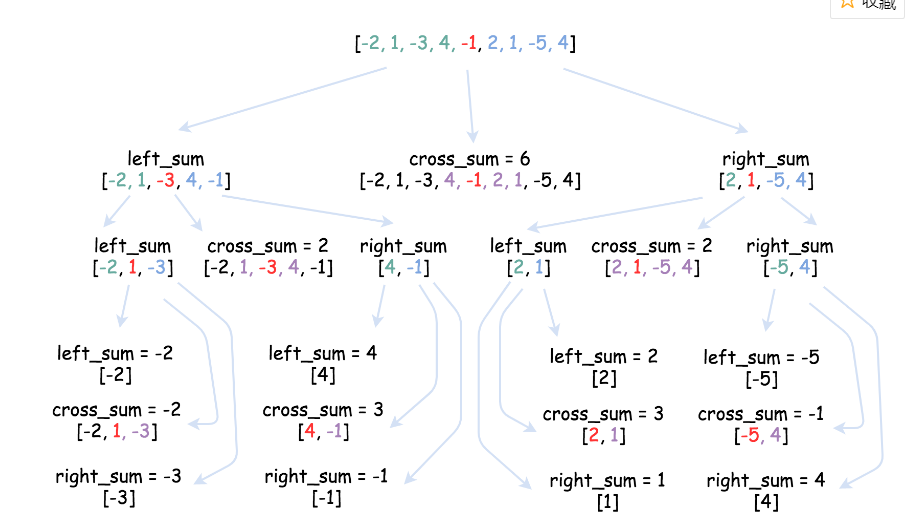代码：
class Solution:
def cross_sum(self, nums, left, right, p):
if left == right:
return nums[left]

left_subsum = float('-inf')
curr_sum = 0
for i in range(p, left - 1, -1):
curr_sum += nums[i]
left_subsum = max(left_subsum, curr_sum)

right_subsum = float('-inf')
curr_sum = 0
for i in range(p + 1, right + 1):
curr_sum += nums[i]
right_subsum = max(right_subsum, curr_sum)

return left_subsum + right_subsum

def helper(self, nums, left, right):
if left == right:
return nums[left]

p = (left + right) // 2

left_sum = self.helper(nums, left, p)
right_sum = self.helper(nums, p + 1, right)
cross_sum = self.cross_sum(nums, left, right, p)

return max(left_sum, right_sum, cross_sum)

def maxSubArray(self, nums):
return self.helper(nums, 0, len(nums) - 1)


题目2：逆序对
在数组中的两个数字，如果前面一个数字大于后面的数字，则这两个数字组成一个逆序对。输入一个数组，求出这个数组中的逆序对的总数。 题解：归并排序：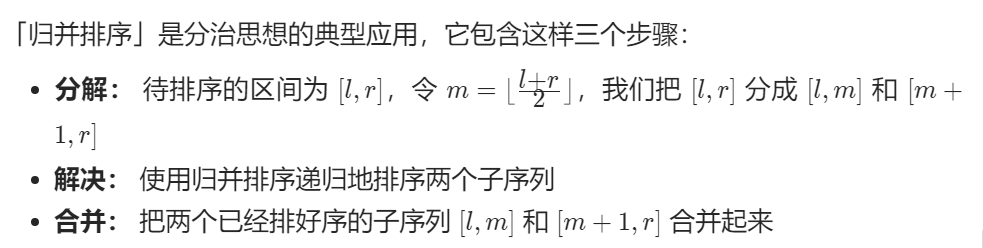如果将数组1【2,3,4,5】与数组2【1,5,6,8】合并，数组1在数组2之前。首先合并2与1中的最小值1。由于2比1大，数组1中的所有数都比1大且都能构成逆序对，逆序对总数加4，以此类推。
class Solution:
def mergeSort(self, nums, tmp, l, r):
if l >= r:
return 0

mid = (l + r) // 2
inv_count = self.mergeSort(nums, tmp, l, mid) + self.mergeSort(nums, tmp, mid + 1, r)
i, j, pos = l, mid + 1, l
while i <= mid and j <= r:
if nums[i] <= nums[j]:
tmp[pos] = nums[i]
i += 1
inv_count += (j - (mid + 1))
else:
tmp[pos] = nums[j]
j += 1
pos += 1
for k in range(i, mid + 1):
tmp[pos] = nums[k]
inv_count += (j - (mid + 1))
pos += 1
for k in range(j, r + 1):
tmp[pos] = nums[k]
pos += 1
nums[l:r+1] = tmp[l:r+1]
return inv_count

def reversePairs(self, nums: List[int]) -> int:
n = len(nums)
tmp =  * n
return self.mergeSort(nums, tmp, 0, n - 1)


展开全文• 分治法相关题目 两个排序数组的中位数 请找出这两个有序数组的中位数。要求算法的时间复杂度为 O(log (m+n)) 。 最大子序和 给定一个整数数组 nums ，找到一个具有最大和的连续子数组（子数组最少包含...
题目汇总
以下链接均为我博客内对应博文，有解题思路和代码，不定时更新补充。
目前范围：Leetcode前150题
分治法相关题目
两个排序数组的中位数

请找出这两个有序数组的中位数。要求算法的时间复杂度为 O(log (m+n)) 。

最大子序和

给定一个整数数组 nums ，找到一个具有最大和的连续子数组（子数组最少包含一个元素），返回其最大和。

最大子序和

将k个排序好的链表合并成新的有序链表

总结
分治算法的基本思想是将一个规模为N的问题分解为K个规模较小的子问题，这些子问题相互独立且与原问题性质相同。求出子问题的解，就可得到原问题的解。即一种分目标完成程序算法，简单问题可用二分法完成。
（1） 分治法基本思想是将一个规模为n的问题分解为k个规模较小的子问题，这些子问题相互独立且与原问题相同。
（2）递归的解这些子问题，然后将各子问题的解合并得到原问题的解。
补充：大数相乘
大数乘法问题及其高效算法：
https://blog.csdn.net/u010983881/article/details/77503519
模拟小学乘法：最简单的乘法竖式手算的累加型；分治乘法：最简单的是Karatsuba乘法，一般化以后有Toom-Cook乘法；快速傅里叶变换FFT：（为了避免精度问题，可以改用快速数论变换FNTT），时间复杂度O(N lgN lglgN)。具体可参照Schönhage–Strassen algorithm；中国剩余定理：把每个数分解到一些互素的模上，然后每个同余方程对应乘起来就行；Furer’s algorithm：在渐进意义上FNTT还快的算法。不过好像不太实用，本文就不作介绍了。大家可以参考维基百科Fürer’s algorithm
https://blog.csdn.net/jeffleo/article/details/53446095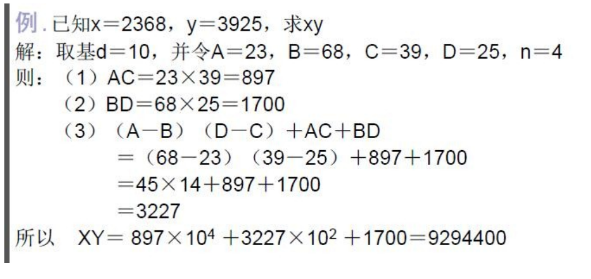展开全文• 题目：一个数列中，排在前面的数比排在后面的数大2倍，称为重要逆序对。计算数列中，重要逆序对的个数。 思路：使用分治算法的归并排序：将数列从中间一分为二，对左边排序；对右边排序；左右使用两个指针，将小的...
3A:重要逆序对
http://algorithm.openjudge.cn/2020hw1/3A/
题目：一个数列中，排在前面的数比排在后面的数大2倍，称为重要逆序对。计算数列中，重要逆序对的个数。思路：使用分治算法的归并排序：将数列从中间一分为二，对左边排序；对右边排序；左右使用两个指针，将小的存入b数组，当右边比左边小时，二分查找左边比右边大2倍的数的位置。
#include<iostream>
#include<cstdio>
#include<cstring>
#include<algorithm>
#include<cmath>
using namespace std;

const int maxn = 200010;
int a[maxn], b[maxn];
int n;
long long ans;

void mergeSort(int low, int high);
void merge(int low, int mid, int high);
int binarySearch(int l, int r, int key);

int main(){
while (cin >> n&&n){
//初始化
ans = 0;
//输入
for (int i = 0; i < n; i++)
cin >> a[i];
//分治
mergeSort(0, n-1);
//输出
printf("%lld\n", ans);
}
return 0;
}

int binarySearch(int l,int r,int key){
int p=r+1;
while (l <= r){
if (l == r){
if (a[l]>key)
p = l;
break;
}
int mid = (l + r) >> 1;
if (a[mid] > key)
r = mid;
else
l = mid + 1;
}
return p;
}

void merge(int low, int mid, int high){
int i = low, j = mid + 1,k=0;
while (k < high - low + 1){
if (i > mid)
b[k++] = a[j++];
else if (j>high)
b[k++] = a[i++];
else{
if (a[i] > a[j]){
ans += mid + 1 - binarySearch(i, mid, 2 * a[j]);
b[k++] = a[j++];
}
else
b[k++] = a[i++];
}
}
for (int i = 0; i < k; i++)
a[i + low] = b[i];
}
void mergeSort(int low, int high){
if (low == high)
return;
int mid = (low + high) >> 1;
mergeSort(low, mid);
mergeSort(mid+1, high);
merge(low, mid, high);
}

3B:Raid
http://algorithm.openjudge.cn/2020hw1/3B/
题目：计算点集1和点集2中两个点最近距离。思路：先对所有点x坐标排序，(只有一个点距离记为∞，有两个点若不同记为这两个点距离，相同记为∞)。找左半部分点的最近距离d1，右半部分点最近距离d2，记d=min(d1,d2)，找所有x距离中间点小于等于d的点，对这些点y坐标排序，两两比较得到最短距离。
#include<iostream>
#include<cstdio>
#include<cmath>
#include<cstring>
#include<algorithm>
using namespace std;

const int maxn = 2010;
const int inf = 0x3f3f3f3f;
struct Point{
int x, y;
int g;//0 or 1
}p[maxn],p1[maxn];
int t, n;

bool cmp_x(Point a, Point b){
return a.x < b.x;
}
bool cmp_y(Point a, Point b){
return a.y < b.y;
}
double dis(Point a, Point b){
return sqrt(pow(a.x - b.x, 2) + pow(a.y - b.y, 2));
}
int absol(int x){
return x>0 ? x : -x;
}
double close_pair(int low, int high){
int k = 0;
if (low == high)
return inf;
if (low + 1 == high){
if (p[low].g != p[high].g)
return dis(p[low], p[high]);
return inf;
}
int mid = (low + high) >> 1;
double d1 = close_pair(low, mid);
double d2 = close_pair(mid+1,high);
double d = min(d1, d2);
for (int i = 1; i <= 2 * n; i++){
if (absol(p[i].x - p[mid].x) <= d)
p1[k++] = p[i];
}
sort(p1, p1 + k, cmp_y);
for (int i = 0; i < k; i++){
for (int j = i + 1; j < k; j++){
if (p1[i].g != p1[j].g){
d = min(d, dis(p1[i], p1[j]));
}
}
}
return d;
}
int main(){
cin >> t;
while (t--){
cin >> n;
for (int i = 1; i <= n; i++){
cin >> p[i].x >> p[i].y;
p[i].g = 0;
}
for (int i = n+1; i <= 2*n; i++){
cin >> p[i].x >> p[i].y;
p[i].g = 1;
}
sort(p + 1, p + 2 * n + 1, cmp_x);
printf("%.3lf\n",close_pair(1, 2 * n));
}
return 0;
}

展开全文• 分治法求最大子段和：利用分治算法先划分为若干个子问题，递归求解每一个子问题，最后将子问题合并，从而求解到整个问题的解。 2.主要数据结构及其作用 一维数组：存储并记录数据 3.测试用例： 4.实验结果截图： ...
1.算法基本思想 分治法求最大子段和：利用分治算法先划分为若干个子问题，递归求解每一个子问题，最后将子问题合并，从而求解到整个问题的解。 2.主要数据结构及其作用 一维数组：存储并记录数据 3.测试用例：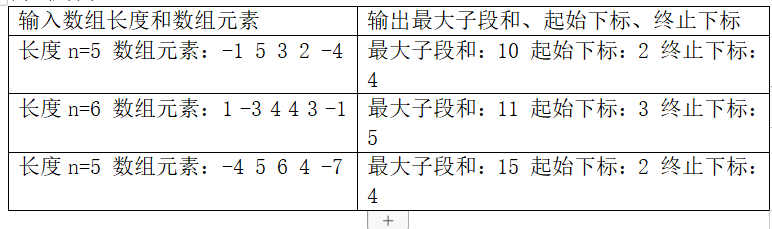4.实验结果截图：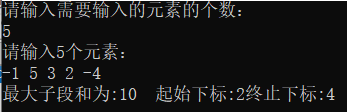测试用例1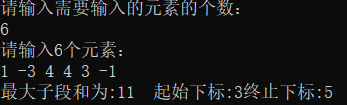测试用例2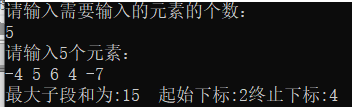测试用例3 5.代码实现
#include<iostream>
using namespace std;
int besti,bestj;
int MaxSubSum(int *a,int left,int right)
{

int sum=0;
if(left==right){
sum=a[left]>0?a[left]:0;
besti=left;
bestj=right;
}

else
{
int center=(left+right)/2;
int leftsum=MaxSubSum(a,left,center);
int rightsum=MaxSubSum(a,center+1,right);
int s1=0;int lefts=0;
for(int i=center; i>=left; i--)
{
lefts+=a[i];
if(lefts>s1){
s1=lefts;
besti=i;
}

}
int s2=0;int rights=0;
for(int i=center+1; i<=right; i++)
{
rights+=a[i];
if(rights>s2){
s2=rights;
bestj=i;
}

}
sum=s1+s2;
if(sum<leftsum)sum=leftsum;
if(sum<rightsum)sum=rightsum;
}
return sum;
}
int main()
{
int n;
cout<<"请输入需要输入的元素的个数："<<endl;
cin>>n;
int a[n];
cout<<"请输入"<<n<<"个元素："<<endl;
for(int i=0; i<n; i++)
cin>>a[i];
cout<<"最大子段和为:"<<MaxSubSum(a, 0, n-1)<<"  ";
cout<<"起始下标:"<<besti+1<<"终止下标:"<<bestj+1<<endl;
}

展开全文数据结构 算法
• ## 分治法及经典例题

千次阅读 2020-04-05 15:17:20
分治法的基本思想 将一个难以直接解决的大问题，分割成一些规模较小的相同问题，以便各个击破，分而治之。 分治法的求解过程： ①划分：将整个问题划分为多个子问题，子问题与原问题有相同的类型。 ②求解：求解各...
• 对于短路的你，希望算法代码给你一个新的思路，代码的讲解利于你更好的对于题目的详细解释同时学会方法，利于自身的创新
• 题目链接：http://acm.zju.edu.cn/onlinejudge/showProblem.do?problemId=5864 题意： 已知K 和 M，满足K在1~N的字典序排列中，处于第M位，求N的最小值。 比如K =2 ，M = 4 的情况，N的最小值为11； 1到11 ...acm
• 题目描述： 给定有n个整数(可能为负整数)组成的序列a1,a2,...,an,求该序列连续的子段和的最大值。 如果该子段的所有元素和是负整数时定义其最大子段和为0。 输入 第一行有一个正整数n(n<1000)，后面跟n个整数,...
• 利用分治法求解凸包问题!c语言 #include #define PPmax 30 #define random(x) (rand()%x) typedef struct node{ float x,y; }Point; Point DingDian[PPmax];//用于存放凸边形的顶点 int DingDnum=0; typedef ...
• 算法思想：分治法 实际问题：最大子段和 编写语言：Java 问题描述 此篇博文是分治法解决最大子段和问题的实现。 问题描述：给定由n个整数(可能为负数)组成的序列A={a1, a2, ..., an}，求该序列形如sum(A, i, j)的...
• 先预排序，预排序后最左和最右的点肯定是凸包中的点。然后可以递归的从内向外扩展凸包，在当前直线的2侧寻找最高点，最高点肯定在凸包中，这里涉及到一些数学知识： a，首先定义射线p1到p2的左侧：若p1 p2 p构成的...
• ## 分治法习题整理

千次阅读 2019-01-01 20:28:56
分治法 1.POJ3714 题目： The system was charged by N nuclear power stations and breaking down any of them would disable the system. The general soon started a raid to the stations by N special agents ...
• 本资源为文档类型，内有相关代码，题目是用分治法解平面最近点对问题，希望有帮助！
• 题目的意思大致是在一个n*m的二维数组中，找到一个局部峰值。峰值要求大于相邻的四个元素（数组边界以外视为负无穷），比如最后我们找到峰值A[j][i]，则有A[j][i] > A[j+1][i] && A[j][i] > A[j-1][i] && A[j][i] > ...
• 与本题相同的题目： 剑指offer42.连续子数组的最大和 <方法一>：分治算法 class Solution { public class Status { public int lSum, rSum, mSum, iSum; public Status(int lSum, int rSuleetcode
• Tags: 分治法设p1=(x1, y1), p2=(x2, y2), …, pn=(xn, yn)是平面上n个点构成的集合S，设计算法找出集合S中距离最近的点对。输入多组测试数据，第一行为测试数据组数n（0&lt;n≤100），每组测试数据由两个部分...
• LeetCode刷题-分治法概述例题多种元素三级目录 概述 例题 多种元素 三级目录leetcode
• 分治法解决 算科学 目 最近距离 问题2.分 支限界解决旅行商 售货员问题 评 语 组长签字 成 绩 I 期 20 年 月 课程设计任务书 学 院 理学院 专 信息与计算科学 业 学生姓 xx 班级 xx 名 学号 课程设计 1.分治法解决...
• 递归与分治策略描述的思想是将一个难以直接解决的大问题分割成一些规模较小的相同问题，以便各个击破，分而治之。以下简要介绍几种运用该方法解决的著名问题，以备学习复习笔试面试之用。这些内容包括： 1.汉诺塔...算法整理
• 分治法的经典题目 50. Pow(x, n) 53. 最大子序和 169. 多数元素 分治法简介 分治法，即“分而治之”，就是将原问题分解为几个规模较小但是类似于原问题的子问题，递归求解这些子问题， 然后再合并这些问题的解...算法 数据结构 python
• 分治法思想 　将原问题分解为几个规模较小但类似于原问题的子问题,递归地求解这些子问题,然后再合并这些子问题的解来建立原问题的解. 分治模式在每层递归时都有三个步骤: 　分解原问题为若干子问题,这些子问题...
• 分治法解最近对问题 问题地址 [洛谷](<https://www.luogu.org/problem/P1257) 题目描述 Description 最近对问题：使用分治算法解决最近对问题。 Input 第一行为测试用例个数。后面每一行表示一个用例，一个用例为...
• ## 分治法求众数

千次阅读 多人点赞 2020-11-08 10:29:52
分治法求众数 Problem Description 给定含有n个元素的多重集合S，每个元素在S中出现的次数称为该元素的重数。多重集S中重数最大的元素称为 众数。例如，S={1，2，2，2，3，5}。多重集S的众数是2，其重数为3。 求...数据结构 算法
• 分治法是一种很重要的算法。字面上的解释是“分而治之”，就是把一个复杂的问题分成两个或更多的相同或相似的子问题，再把子问题分成更小的子问题……直到最后子问题可以简单的直接求解，原问题的解即子问题的解的...python 算法
• 分治法算法分析作业 吴迪 2011-3-29 本次是第一次算法作业该部分内容包含 3 个题目的程序代码分析文档说明图片等 内容 . 目录 引言 3 1 算法性能比较 3 1.1 问题分析 3 1.2 源程序代码 3 1.3 运行示例 8 1.4 数据...
• 使用快速排序算法实现对n个元素进行排序。 由文件input.txt提供输入数据，输出到文件output.txt中。快速排序
• ## 分治法-合并排序

千次阅读 多人点赞 2019-08-07 20:13:02
合并排序用到了分治策略实现对元素进行排序。 合并排序的基本思想：把待排序的n个元素分解成n组，也就是每组一个元素；之后对分好的组进行两两合并（无配对的则不操作），以此类推。 以序列{8, 3, 2, 6, 7, 1, 5, ...合并排序
• 分治法 主要思想：将一个复杂问题分成若干个相同或相似的子问题递归求解，最后将子问题的解合并 divide-conquer 对应题目已用java实现 分治法主要利用递归来实现，分治法和递归都是DFS深度优先搜索的思想。 分治法...算法
• 使用分治算法，求一个数组中的最大数和最小数。 Input 多组数据输入，每组第一个数字为数组的长度n, 然后接下输入n个整数 Output 依次输出数组中的最大值与最小值 样例输入输出 样例输入 5 1 5 2 4 3 6 1 2 3 4 5 6 ...算法 c++ c语言...